#### Using the original action variables

Once the CFS equation has been determined, the problem is almost solved. The action trajectory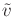was determined from the given state trajectoryand the backward P. Hall coordinate trajectory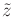is determined by (15.143). The only remaining problem is that the action variables from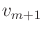to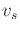are fictitious because their associated vector fields are not part of the system. They were instead obtained from Lie bracket operations. When these are applied, they interfere with each other because many of them may try to use the samevariables from the original system at the same time.

The CBHD formula is used to determine the solution in terms of the system action variables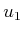,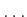,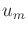. The differential equation now becomes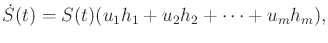(15.144)

which is initialized with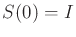and uses the original system instead of the extended system.

When applying vector fields over time, the CBHD formula becomes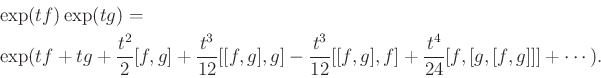(15.145)

If the system is nilpotent, then this series is truncated, and the exact effect of sequentially combining constant motion primitives can be determined. This leads to a procedure for determining a finite sequence of constant motion primitives that generate a motion in the same direction as prescribed by the extended system and the action trajectory.

Steven M LaValle 2020-08-14